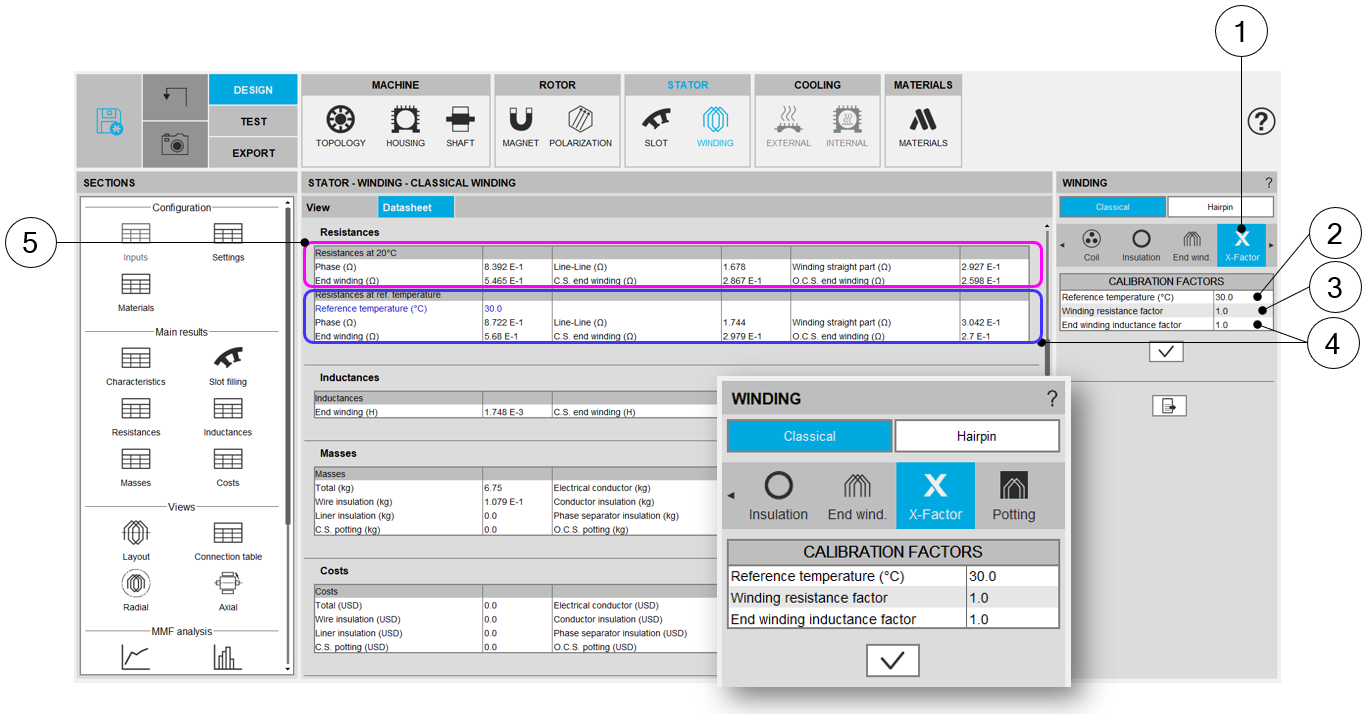# Calibration factors

## 1. Overview - Definitions

 Label Symbol Tooltip, note, formula Resistance factor * Setting of the “Resistance factor”: It allows modifying the computation result of resistance. Thus, the resulting phase resistance value is considered. Inductance factor * Setting of the “Inductance factor”. It allows modifying the computation result of end-winding inductance. Thus, the resulting end-winding inductance value is considered. Ref. temperature * The reference temperature: First, the resistance values are computed by considering a temperature equal to 20°C. However, the user can also define his own reference temperature to compute the corresponding phase resistance and Line-Line resistance values. Note: This reference temperature is used only in the winding design environment.The test temperatures are defined in the test settings (refer to TEST chapter).

## 2. IllustrationsBuilding the winding – X-Factor = Calibration factors 1 Selection of the X-FACTOR section. 2 Setting of the “Resistance factor”. It allows adjusting computation result of resistance. Thus, the resulting phase resistance value is considered. 3 Setting of the “Inductance factor”. It allows modifying the computation result of end-winding inductance. Thus, the resulting end-winding inductance value is considered. 4 The reference temperature: First, resistance values are computed by considering a temperature equal to 20°C (5). However, the users can also define their own reference temperature to compute the corresponding phase resistance and Line-Line resistance values. 5 Resistance values for a reference temperature equal to 20°C.